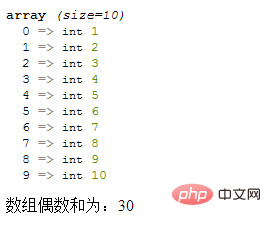# PHP数组怎么根据指定条件求和PHP数组根据指定条件求和

`\$sum=0;`

```foreach (\$arr as \$v){
//循环体语句块;
}```

```if(条件){
\$sum+=\$v;
}```

```<?php
\$arr = [1,2,3,4,5,6,7,8,9,10];
var_dump(\$arr);
\$sum=0;
foreach(\$arr as \$v){
if(\$v%2==0){
\$sum+=\$v;
}
}
echo "数组偶数和为：".\$sum;
?>```• 相关标签：php php数组
• 相关文章

相关视频